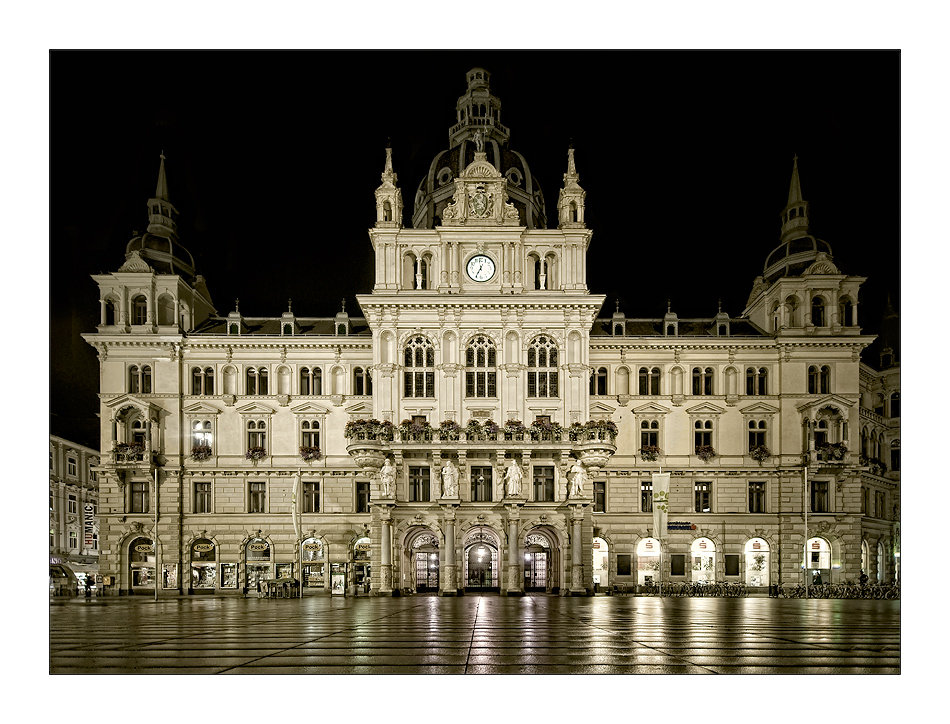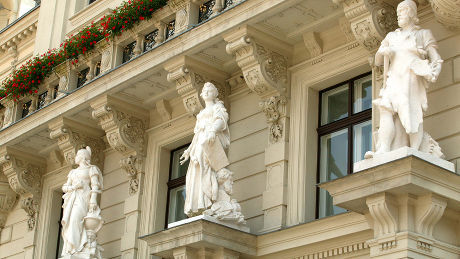# Pure Quintic FieldsOur Mission:

to the Forefront of International Research
and to stabilize this statusPure Quintic Fields § 1. Galois closure and subfield lattice. Let q1,…,qs be pairwise distinct primes such that s ≥ 1 and 5 may be among them. Denote by L = Q(D1/5) the pure quintic number field with fifth power free radicand D = q1e1 … qses, where the exponents are integers 1 ≤ ej ≤ 4. The field L is generated by adjoining the unique real solution of the pure quintic equation X5 - D = 0 to the rational number field Q. It is a non-Galois algebraic number field with signature (1,2) and thus possesses four isomorphic complex fields Lj = Q(ζjD1/5), 1 ≤ j ≤ 4, all of whose arithmetical invariants coincide. The normal closure of L is the compositum N = Q(D1/5,ζ) of L = Q(D1/5) with the cyclotomic field k = Q(ζ), where ζ = ζ5 = exp(2 π i / 5) denotes a primitive fifth root of unity. N is a complex metacyclic field of degree 20 whose Galois group Gal(N|Q) is the semidirect product M5 = C5 * C4 of two cyclic groups. The cyclotomic field k is a complex cyclic quartic field and contains the real quadratic field Q(51/2) as its maximal real subfield k+ = Q(ζ + ζ-1). Consequently, there exists a real intermediate field M = Q(D1/5,51/2) of degree 10 between L and N, which is non-Galois with signature (2,4). § 2. Class numbers and unit index. In 1973, Parry has determined the class number relation 55 hN = (UN:U0) hL4 [Thm. I, p. 476, Pa], where UN denotes the unit group of N, U0 is the subgroup of UN generated by all units of the conjugate fields Lj = Q(ζjD1/5), 0 ≤ j ≤ 4, of L and of k, and the unit index (UN:U0) can take seven possible values 5e with 0 ≤ e ≤ 6 [Thm. II, p. 478, Pa]. Examples 2.1. Anticipating some of our numerical results in section 8, we give the smallest radicands D of pure quintic fields L = Q(D1/5) where the various values of the exponent e actually occur: e = 6 for D = 6 = 2*3 of type γ, e = 5 for D = 2 of type ε, e = 4 for D = 22 = 2*11 of type β2, e = 3 for D = 11 of type α2, e = 2 for D = 31 of type α1, e = 1 for D = 33 = 3*11 of type α2. However, we point out that we did not find a realization of e = 0, that is, UN is never generated completely by subfield units. At the end of his article, Parry suggests verbatim "In conclusion the author would like to say that he believes a numerical study of pure quintic fields would be most interesting" [p. 484, Pa]. Of course, it would have been rather difficult to realize Parry's desire in 1973. But now, 40 years later, we are in the position to use the powerful computer algebra systems PARI/GP and MAGMA to start an attack against this hard problem. This will be done in our present research line. Next Page